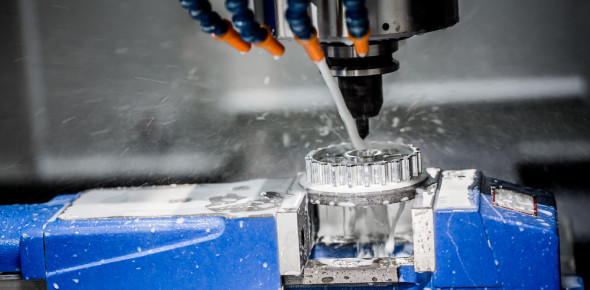# Quiz About Iron And Steel Manufacturing!

25 QuestionsSettingsThe iron and steel Industry is the basic industry since all the other industries — heavy, medium and light, depend on it for their machinery. Steel is needed to manufacture a variety of engineering goods, construction material, defence, medical, telephonic, scientific equipment and a variety of consumer goods. This is a Quiz about Iron and Steel Manufacturing!

• 1.
What is the name of work holding device were the irregular work could hold in lathe?
• A.

Face plate

• B.

Catch plate

• C.

B-Jaw chuck

• D.

Driving place

• 2.
What is angle between the two faces of angle plate?
• A.

30° 90°

• B.

45°

• C.

60°

• D.

90°

• 3.
Which material is used to make angle plate?
• A.

Mild steel

• B.

Cast iron

• C.

Tool steel

• D.

Wrought iron

• 4.
What does the letter ‘a’ denotes?
• A.

Sampling length

• B.

Roughness values

• C.

Production method

• D.

Machining allowance

• 5.
How the roughness value is expressed?
• A.

Micrometer

• B.

Millimetre

• C.

Centimetre

• D.

Metre

• 6.
What is the roughness grade number for symbol?
• A.

N1 to N3

• B.

N4 to N6

• C.

N11

• D.

N12

• 7.
What is the surface roughness symbol marked as ‘X’?
• A.

Direction of lay

• B.

Sampling length

• C.

Machining allowance

• D.

Roughness value

• 8.
What is the roughnes value of grade No N1?
• A.

6.3 microns

• B.

0.025 microns

• C.

12.5 microns

• D.

0.4 microns

• 9.
Which material used for manufacturing a marking table?
• A.

Cast iron

• B.

Aluminium

• C.

Tool steel

• D.

Mild steel

• 10.
What is the value of “b” in the surface roughness symbol?
• A.

• B.

Sampling length

• C.

Production method

• D.

Direction of lay

• 11.
What is the type of angle plate?
• A.

Plain

• B.

Box

• C.

Swivel

• D.

Slotted

• 12.
What is the type of angle plate?
• A.

Plain

• B.

Slotted

• C.

Box

• D.

Swivel

• 13.
What is the name of part marked as ‘x’?
• A.

Counter weight

• B.

Face plate

• C.

Work piece

• D.

Angle plate

• 14.
What is lapping?
• A.

Precision finishing operation

• B.

Filing operation

• C.

Grinding operation

• D.

Chiseling operation

• 15.
How may roughness grade number are used?
• A.

N1 to N12

• B.

N1 to N25

• C.

N1 to N20

• D.

N1 to N30

• 16.
Which lapping material used for easy charging and rapid cutting?
• A.

Cast iron

• B.

Copper and brass

• C.

Close grained iron

• D.

White cast iron

• 17.
What is the roughness grade number for symbol?
• A.

N7 to N9

• B.

N4 to N6

• C.

N1 to N3

• D.

N12

• 18.
What is the name of device marked as ‘X’?
• A.

Lathe plate

• B.

Four jaw chuck

• C.

Faceplate

• D.

Driving plate

• 19.
Which grade of angle plate are more accuracy and used for tool room work?
• A.

• B.

• C.

• D.

• 20.
Which lathe part is used to mount the dial indicator for truing the bore?
• A.

Tail stock

• B.

Cross side

• C.

Tool post

• D.

Lathe bed

• 21.
What is the operation?
• A.

Checking angles

• B.

Checking the squareness

• C.

Checking for height

• D.

Checking for parallelism

• 22.
How the cutting speed is expressed?
• A.

Foot/min

• B.

Meter/sec

• C.

Meter/min

• D.

Revoluation/min

• 23.
What is the length of the tool travelled in one revolution if the diamenter of work is ‘D’?
• A.

2 pi

• B.

Pi r 2

• C.

Pi x D

• D.

4 pi r 2

• 24.
What is the recommended cutting speed for turning mild steel using H.S.S tool?
• A.

25-40 m/min

• B.

35-70 m/min

• C.

40-70 m/min

• D.

35-50 m/min

• 25.
What is the term marked as ‘x’?
• A.

Diameter over roller at smaller end

• B.

Diameter over roller at bigger end

• C.

Height of slip gauge

• D.

Height of the job

Related TopicsBack to top